# PHY4: CURRENT, EM AND RESISTANCE

##### This unit is about resistance and current in electricity.

CURRENT, EMF AND RESISTANCE

A simple electrical circuit is shown below with a battery connected to a resistor.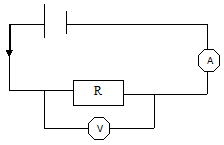QUANTITY MEASUREMENT INSTRUMENT UNIT SYMBOL CURRENT AMMETER AMPERE A POTENTIAL DIFFERENCE VOLTMETER VOLTS V EMF VOLTMETER VOLTS V RESISTANCE RHEOSTAT OHMS Ω

Ammeter/Voltmeter usage

A voltmeter is always connected in parallel with the component whose p.d it is supposed to measure. It is constructed with a high resistance so that current does not pass through it.

The ammeter is connected in series with a component whose current is required. It should have low resistance compared with the rest of the circuit so that it does not introduce unwanted resistance.

The Coulomb

The coulomb is the unit of quantity of electricity.

The coulomb is the quantity of electricity that passes any point in an electrical circuit in one second when a steady current flowing through it is one ampere.

Therefore

Quantity of electricity= current x time

Q=It

The Volt

The volt is the potential difference between two points if the work done to transfer one coulomb of charge from one point to the other is one joule.

Potential difference [p.d]

If a negatively charged conductor is connected to the earth, electrons flow from the conductor to the earth. If a positively charged conductor is connected to the earth, electrons flow from the earth to the conductor.

We think of p.d as being the electrical condition which governs the direction of flow of electricity from one point to another.

Potential difference is analogous to temperature difference that causes flow of heat between two points or pressure difference that causes flow of liquid.

The potential difference between two points is the work done in moving one coulomb of positive charge from one point to the other.

Earth at Zero Potential

The earth is considered to be at zero potential. This does not mean the earth has no electrical charge. All points on its surface are at the same potential.

Electromotive force

The e.m.f is sum of potential differences which it can produce across all the various components of a circuit in which it is connected including the potential difference required to drive a current through the cell itself.

The electromotive force is defined as the total work done per coulomb of electricity conveyed in a circuit in which the cell is connected.

RESISTANCE
The resistance of a conductor is the ratio of the potential difference across it to the current flowing through it.

A good conductor has low resistance and a poor conductor has high resistance to current flow.

Resistance of a conductor such as a wire depends on its length, cross-sectional area and material from which it is made.

Silver offers least resistance to current flow but is expensive, so copper is preferred in making wires for electrical conduction.

Common symbols in electric circuit diagrams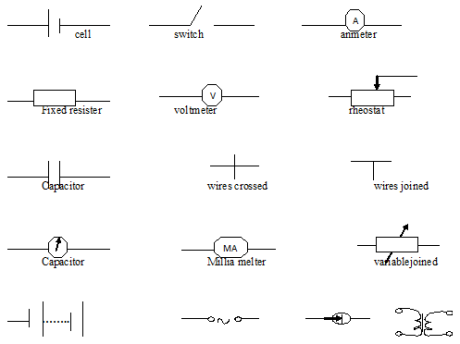Effect of temperature on resistance

• The resistance of a pure metal increases with temperature but the resistance of certain other conductors such as carbon decreases with increase in temperature.
• The resistance of semiconductors decreases with rising temperature e.g. germanium, silicon.

Factors affecting the resistance of a wire at constant temperature

• Length —the greater the length the higher the resistance.
• Area –If the area of a conductor increases its resistance decreases.
• Nature of the material of the wire.

The three factors can be expressed in form of an equation

R= rl/a

The quantity is called the resistivity of a material of the wire.

Units of p are Ωm. It is also a constant.

THIS VIDEO SHOWS YOU MORE ABOUT CURRENT

OHM’S LAW

The current passing through a conductor at constant temperature is proportional to the potential difference between its ends.

(p.d) V=IR

The ohm is the resistance of a conductor such that when a potential difference of one
volt is applied to its ends current of one ampere flows through it.

Limitations of ohms law

• It does not apply to semiconductors
• It does not apply to conduction of electricity through gases
• All physical conditions must remain constant.

Arrangement of resistors

• Series arrangementThe resistors are connected end to end.

The same current flows through all of them.

Since V = IR

The total potential difference is the sum of individual potential differences across
R1, R2, R3,

V=V1 + V2 + V3

V1=IR1, V2 = IR2, V3 =IR3

V=IR1+ IR2 + IR3

V=I (R1+ R2 + R3)

V/I = R1 + R2 + R3

But V/I  =R

Where R is the total resistance

R = R1 + R2 + R3

Parallel arrangement of resistors

Resistors are said to be in parallel when their corresponding ends are joined together.

The same p.d is thus applied to each.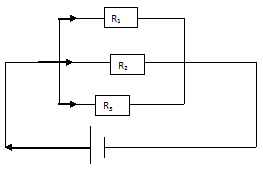Current I = I1 + I2 + I3

V = I1RI V = I2R2V = I3R3Arrangement of cells

Series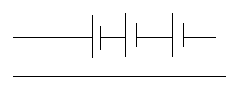The total emf of a battery is the sum of the separate emf’s and the internal resistance is ‘ equal to the sum of internal resistances of the cells.

ParallelIf the cells have equal emf and internal resistance the resultant emf is the same as that of one cell.

The internal resistance is obtained using expression for resistors in parallel.

Advantage of connecting cells in parallel

• There’s less drain on the cells since they share the total current whereas with series
connection the same main current is supplied by each cell.

An experiment to measure the internal resistance of a cell

Connect a high resistance voltmeter, to the cell terminals and record its reading as E.

Connect the cell to a resistance box and voltmeter as shown.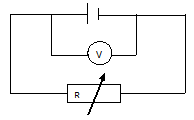Note the voltmeter reading when the resistance R is adjusted to lΩ,2Ω,3Ω,4Ω

Record the results in a table including values of r where r = R[E-V] / V

 R/Ω V/V r/Ω I 2 3 4

Theory of experiment

If is the internal resistance of the cell E =I(R+ r)

= IR+Ir

= IR  , E = V+ Ir

E-V / I

r = R[E-V] / V

The average value of r is then calculated and that is the internal resistance of the cell.

‘Lost volts’

When a cell has got internal resistance, P.d is required to drive a current through the cell itself this p.d is called the lost volts.

‘Lost volts’ is the potential difference required to drive a current across the terminals of a cell that has got internal resistance.

Terminal P.d

It is the potential difference required to send the current through the external resistor. Terminal p.d is always less than the emf and the difference between them is the ‘lost volts’.

Calculations/examples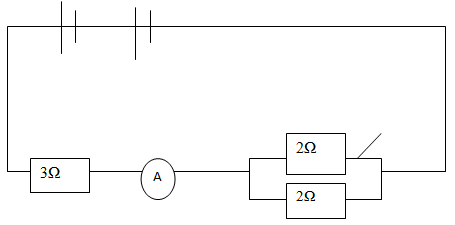In the above circuit find the ammeter reading when the switch is

1. Open
2. Closed assuming the battery has negligible internal resistance.

Solution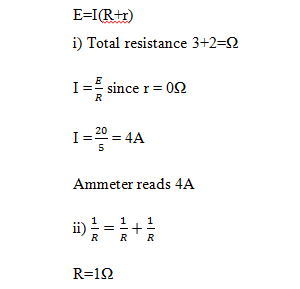A cell C supplies current to the 3Ω and 2Ω resistors as shown. The current in the 3Ω resistor is 1.2A. Find

1. The current in the 2 resistor.
2. the current I
3. P.d of cell C

Solution
p.d across 3Ω resistor = 1.2×3=3.6V

p.d across 2Ω resistor = p.d across 3Ω resistor=3.6.

1.I2 = 3.6/2  = 1.8A

2.I = I1+I2

But I1=1.2A

I=1.2+1.8=3A

(iii) p.d of cell C= 3.6V

## STEM Elearning

We at FAWE have built this platform to aid learners, trainers and mentors get practical help with content, an interactive platform and tools to power their teaching and learning of STEM subjects, more

#### How to find your voice as a woman in Africa

© FAWE, Powered by: Yaaka DN.